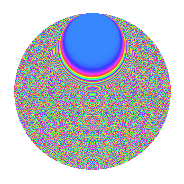# Properties

 Label 1350.2.biLevel 1350 Weight 2 Character orbit bi Rep. character $$\chi_{1350}(23,\cdot)$$ Character field $$\Q(\zeta_{180})$$ Dimension 4320 Sturm bound 540

# Related objects

## Defining parameters

 Level: $$N$$ = $$1350 = 2 \cdot 3^{3} \cdot 5^{2}$$ Weight: $$k$$ = $$2$$ Character orbit: $$[\chi]$$ = 1350.bi (of order $$180$$ and degree $$48$$) Character conductor: $$\operatorname{cond}(\chi)$$ = $$675$$ Character field: $$\Q(\zeta_{180})$$ Sturm bound: $$540$$

## Dimensions

The following table gives the dimensions of various subspaces of $$M_{2}(1350, [\chi])$$.

Total New Old
Modular forms 13152 4320 8832
Cusp forms 12768 4320 8448
Eisenstein series 384 0 384

## Trace form

 $$4320q + O(q^{10})$$ $$4320q + 24q^{20} - 24q^{23} + 144q^{25} - 12q^{27} + 72q^{30} + 12q^{33} + 108q^{35} + 36q^{38} + 48q^{42} + 60q^{45} + 48q^{47} + 12q^{48} + 48q^{50} + 36q^{57} + 60q^{59} - 84q^{63} - 24q^{65} + 72q^{67} + 144q^{68} + 48q^{72} + 36q^{75} - 720q^{77} - 24q^{78} + 60q^{83} + 252q^{87} + 48q^{92} + 96q^{93} + 60q^{95} + 72q^{97} + O(q^{100})$$

## Decomposition of $$S_{2}^{\mathrm{new}}(1350, [\chi])$$ into newform subspaces

The newforms in this space have not yet been added to the LMFDB.

## Decomposition of $$S_{2}^{\mathrm{old}}(1350, [\chi])$$ into lower level spaces

$$S_{2}^{\mathrm{old}}(1350, [\chi]) \cong$$ $$S_{2}^{\mathrm{new}}(675, [\chi])$$$$^{\oplus 2}$$

## Hecke Characteristic Polynomials

There are no characteristic polynomials of Hecke operators in the database